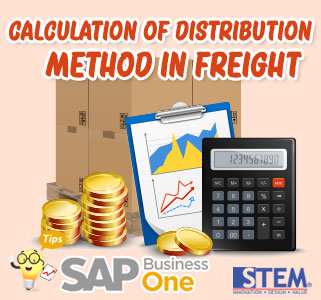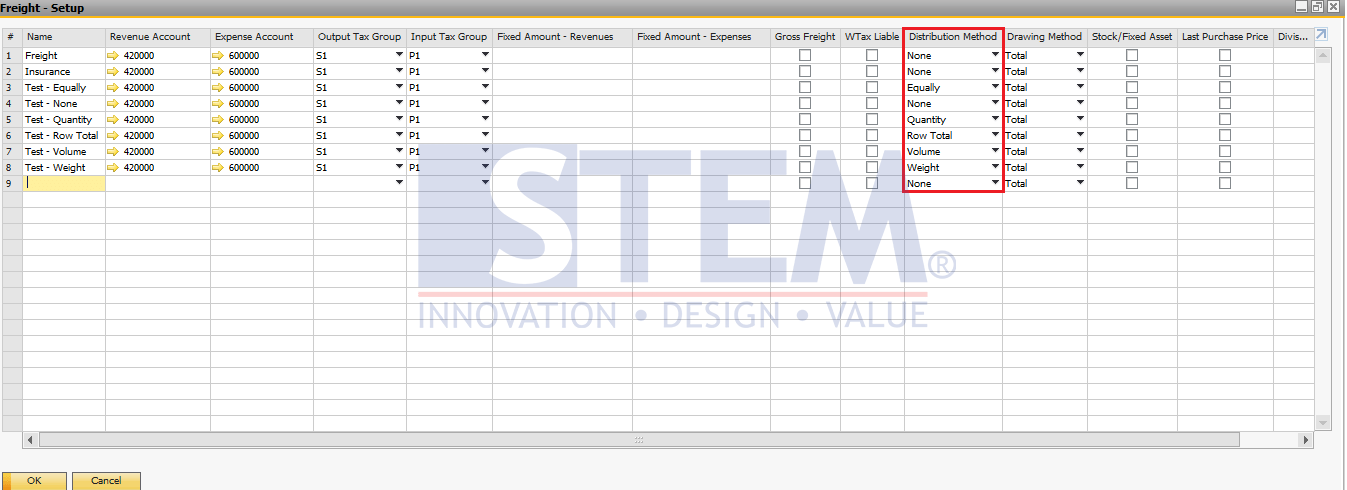PT Sterling Tulus Cemerlang
www.sterling-team.com

Whatsapp
+62-877-8655-5055

Sampoerna Strategic Square
South Tower Level 30
Jl Jend. Sudirman No. 45-46
Jakarta 12930 – Indonesia

500+ FREE Tips for you from Sterling Tulus Cemerlang (STEM) - SAP Indonesia Gold Partner, and Become SAP B1 Super User

# Calculation of Distribution Method in FreightIn the Freight set up, there is a Distribution Method that is useful for choosing a method for distributing Freight values ​​between document rows. Based on your choice, the value of freight is distributed from the header to the line to allocate the Freight to the Quantity or final purchase price (Total Line).SAP Business One Tips – Calculation of Distribution Method in Freight

Distribution Method is divided into 6 of them, namely:

1. None
º The amount of Freight is not distributed between lines in the document details. If you have selected Inventory or Last Purchase Price then this None option cannot be selected.
2. Equally
º Total Freight is distributed evenly for each row in the document details regardless of the value of the Quantity column or the Total of each row
3. Quantity
º The amount of Freight distributed is by looking at the Quantity value for each item’s details.

```Example calculation as follows:
If there are 3 items in a document, including Item 1 Qty = 3, Item 2 Qty = 4, Item 3 Qty = 3. Let us give an example of the value of Freight for the document of \$ 100
Then the distribution of freight calculations will use the formula (Quantity per Item / total items) * Freight Value:
a. Item 1 (3/10) * 100 = \$ 30
b. Item 2 (4/10) * 100 = \$ 40
c. Item 3 (3/10) * 100 = \$ 30```
4. Volume
º The amount of Freight distributed by looking at the Volume value in the document details.

```Example calculation as follows:
If there are 2 items in a document, including Item A Volume = 2000 and Item B Volume = 3000 with a value of \$ 100 Freight
Then the distribution of freight calculations will use the formula (Volume per Item / Total Total Volume) * Freight Value
a. Item A (2000/5000) * 100 = \$ 40
b. Item B (3000/5000) * 100 = \$ 60```
5. Weight
º The amount of Freight is distributed by referring to the weight value if we maintain the weight value in the document details.

```Example calculation as follows:
If in a document there are 2 items, including Item A Weight = 400 and item B Weight 600 with a Freight value of \$ 100
Then the calculation of the Freight calculation will use the formula (Weight per Item / Total Weight) * Freight value
a. Item A (400/1000) * 100 = \$ 40
b. Item B (600/1000) * 100 = \$ 60```
6. Row Total
º The amount of Freight is distributed by referring to the Row Total (Line Total) value of the item in the document item details.

```Example calculation as follows:
If a document contains 2 items, including Item A, Line Total = \$ 40 and item B Line Total \$ 60 with a Freight value of \$ 10
Then the division of the Freight calculation will use the formula (Total Row per Item / Total Total Row Total) * Freight Value
a. Item A (40/100) * 10 = \$ 4
b. Item B (60/100) * 10 = \$ 6```

•List of Object Types On SAP BUSINESS ONE (105,624)
•Restart SAP HANA Services in SuSE Linux (65,752)
•List of Document Type on SAP B1 (47,542)
•How to Solve Failed to Connect SLD Issue (36,035)
•Monitoring Access Log & Change Log for Identify User Access (31,590)